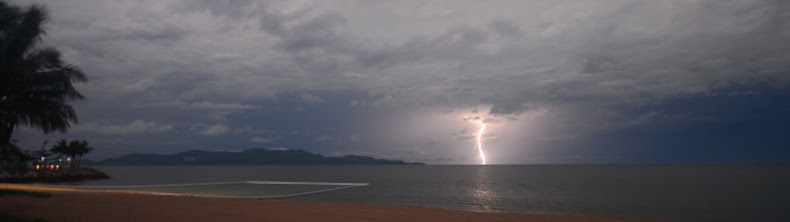Wednesday, January 27, 2010

And now for something on the lighter side:

1. Subtract the most southerly latitude you've visited from the most northerly latitude you've visited (flying over it doesn't count!). Retain the negative sign for southerly latitudes.

2. Subtract the most westerly (i.e., the most negative) longitude from the most easterly longitude you've visited. Retain the negative sign on west longitudes.

3. Subtract the most negative altitude (below sea level, if necessary) from the most positive altitude you've visited. Divide by 10.

4. Add 1, 2, and 3 together.

5. As an alternate fun exercise, instead of subtracting each point, average them instead (this time, add instead of subtract so that the negatives offset the positives), and find out where the resulting point (latitude, longitude, altitude) puts you on the earth. (This website will do it for you)

My results:
1. Most southerly latitude: -43 (Bruny Island, Tasmania)
Most northerly latitude: +64 (Ekati Diamond Mine, NWT)
64-(-43)= 107.
2. Most westerly longitude: -159 (Kauai, Hawaii)
Most easterly longitude: +153 (Brisbane, Queensland)
153-(-159)= 312.
3. Most negative altitude: -40m (Jewel Cave, Western Australia)
Most positive altitude: 2284m (Blackcomb Peak, British Columbia)
2284-(-40)= 2324/10=232
4. 107+312+232= 651

5. (64+[-43])/2=21 N
(153-[-159])/2=156 E
2284+(-40)/2=1122 m
(Somewhere in the West Pacific!)

1.Isn't Greenwich zero degrees longitude? Similarly, isn't Cape Leeuwin easterly rather than westerly?

My numbers:

1. Most southerly latitude: -47 (Stewart Island, NZ)
Most northerly latitude: +53 (Castlebar, Ireland)
53-(-47)=100

2. Most westerly longitude: -172 (Savai'i, Western Samoa)
Most easterly longitude: +178 (Nadi, Fiji)
178-(-172) = 350

3. Most negative altidude: -81 (Badwater, California)
Most positive altitude: 4421 m (Mount Whitney, California)
4421-(-81) = 4502/10 = 450

4. 450+350+100 = 900

5. 53-47=6/2=3N
178-172=6/2=3E
4421-81=4340/2=2170m

Gulf of Guinea, off the coast of Nigeria and Cameroon.

2.Had a total brainfart there . . . you are correct on both counts.